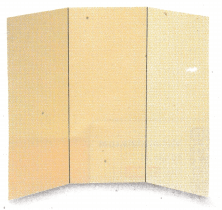# Texas Go Math Grade 7 Module 7 Answer Key Linear Relationships

Refer to our Texas Go Math Grade 7 Answer Key Pdf to score good marks in the exams. Test yourself by practicing the problems from Texas Go Math Grade 7 Module 7 Answer Key Linear Relationships.

## Texas Go Math Grade 7 Module 7 Answer Key Linear Relationships

Evaluate each expression.

Question 1.
2(5) + 11 __________
2(5) + 11 = 10 + 11 Multiply 2 by 5.
= 21 Add 11 to this product.
2(5) + 11 = 21

Question 2.
9(6) – 5 __________
9 (6) – 5 = 54 – 5 Multiply 9 by 6.
= 49 Subtract 5 from this product
9(6) – 5 = 49

Question 3.
4(12) – 15 __________
4 (12) – 15 = 48 – 15 Multiply 4 by 12.
= 33 Subtract 15 from this product.
4(12) – 15 = 33

Question 4.
-6(2) + 13 ___________
-6(2) + 13 = -12 + 13 Multiply -6 by 2.
= 1 Add 13 to this product.
-6(2) + 13 = 1

Question 5.
7(-4) – 8 ____________
7(- 4) – 8 = -28 – 8 Multiply 7 by -4.
= -36 Since both numbers are negative, they are added together with the -“ sign.
7(-4) – 8 = -36

Question 6.
-2(-5) + 7 ____________
-2(- 5) + 7 = 10 + 7 Multiply -2 by -5.
= 17 Add 7 to this product.
-2(-5) + 7 = 17

Find a rule relating the given values.

Question 7.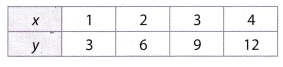Set the equation for x = 1, y = 3. For all the others apply the same rule.
1 ∙ z = 3
Notice that this variable z is 3 because;
1 ∙ 3 = 3
Therefore the rule for the given value is:
y = x ∙ 3

Question 8.Set the equation for x = 1, y = 9. For all the others apply the same rule
1 + z = 9
Subtract one from each side.
z = 1 – 1 + z = 9 – 1 = 8
Therefore the rule for the given value is:
y = x + 8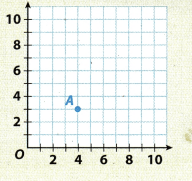Graph point A(4, 3). Start at the origin. Move 4 units right. Then move 3 units up.

Graph each point on the coordinate grid above.

Question 9.
B(9, 0)
For graphing a point A = (9, 0) starting from the origin move 9 units right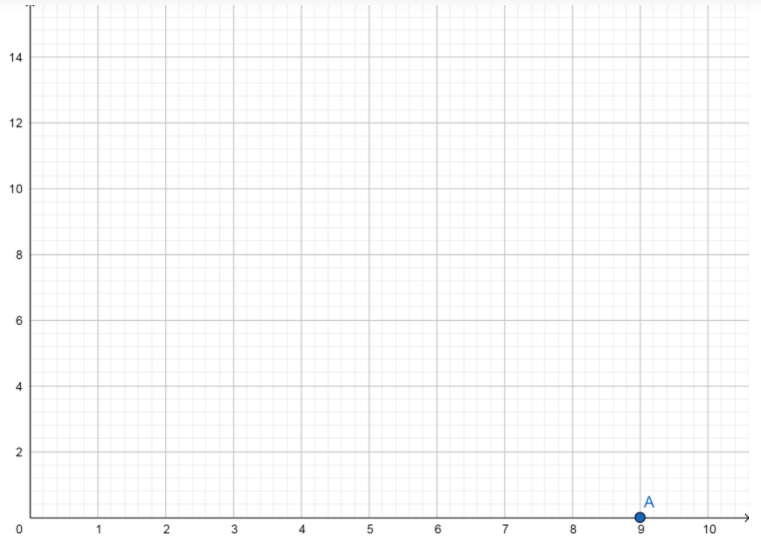Question 10.
C(2, 7)
C = (2, 7)
Starting from the origin move 2 units right and than move 7 units up.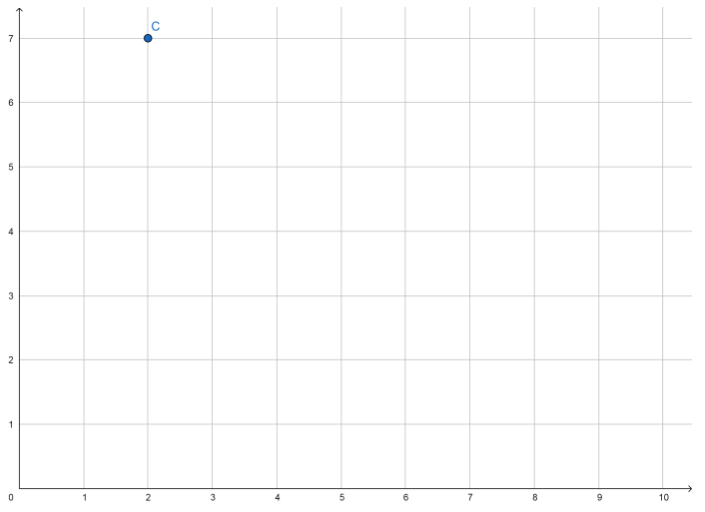Question 11.
D(0, 5)
D = (0, 5)
Starting from the origin move 5 units up.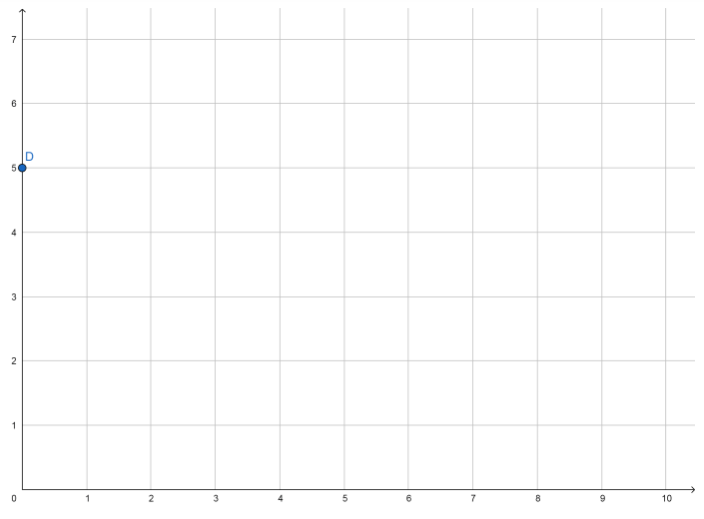Question 12.
E(6, 2)
E = (6, 2)
Starting from the origin move 6 units right and then move 2 units up.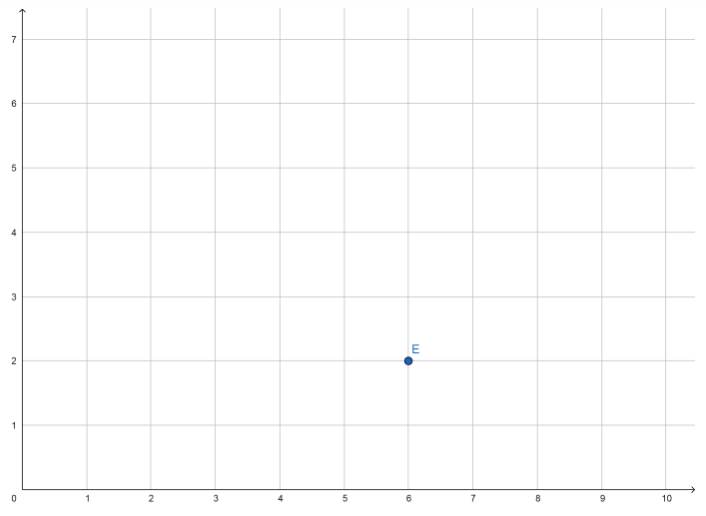Visualize Vocabulary

Use the ✓ words to complete the third column of the chart.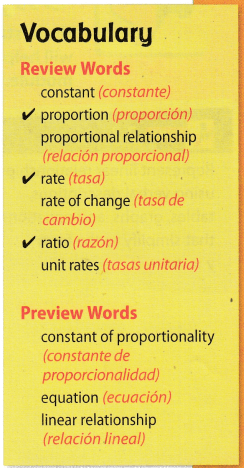Understand Vocabulary

Answer each question with the correct preview word.

Question 1.
What is a mathematical statement that two expressions are equal?